## Definition of Inductance of Transmission Line:

The formula for definition of inductance of transmission line is as follows:

Voltage induced in a circuit is given by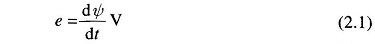where ψ represents the flux linkages of the circuit in weber-turns (Wb-T).

This can be written in the form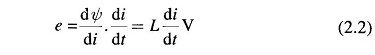where L=dψ/di is defined as the inductance of the circuit in henrys, which in general may be a function of i. In a linear magnetic circuit, i.e., a circuit with constant permeability, flux linkages vary linearly with current such that the Inductance is constant given by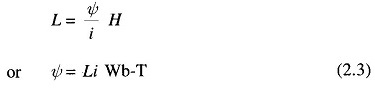If the current is alternating, the above equation can be written as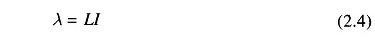where λ and I are the rms values of flux linkages and current respectively.

These are of course in phase.

Replacing d/dt  in Eq. (2.1) by jω, we get the steady state AC voltage drop due to alternating flux linkages as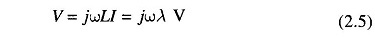On similar lines, the mutual inductance between two circuits is defined as the flux linkages of one circuit due to current in another, i.e.,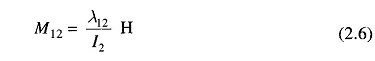The Voltage drop in circuit 1 due to current in circuit 2 is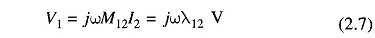The concept of mutual inductance is required while considering the coupling between parallel lines and the influence of power lines on telephone lines.

Scroll to Top